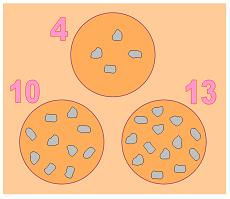#### You may also like### N000ughty Thoughts

How many noughts are at the end of these giant numbers?### Mod 3

Prove that if a^2+b^2 is a multiple of 3 then both a and b are multiples of 3.### Novemberish

a) A four digit number (in base 10) aabb is a perfect square. Discuss ways of systematically finding this number. (b) Prove that 11^{10}-1 is divisible by 100.

# Odd Stones

#### $27$ stones are distributed between $3$ circlesOn a "move" a stone is removed from two of the circles and placed in the third circle.

So, in the illustration, if a stone is removed from the $4$ and the $10$ circles and added to the $13$ circle, the new distribution would be $3$ - $9$ - $15$

#### Check you can turn $2$ - $8$ - $17$ into $3$ - $9$ - $15$ in two "moves"

Here are five of the ways that $27$ stones could be distributed between the three circles :

$6$ - $9$ - $12$

$3$ - $9$ - $15$

$4$ - $10$ - $13$

$4$ - $9$ - $14$

$2$ - $8$ - $17$

There is always some sequence of "moves" that will turn each distribution into any of the others - apart from one.

Identify the distribution that does not belong with the other four.

Can you be certain that this is actually impossible rather than just hard and so far unsuccessful?